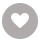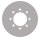# Python: Deeper Insights into Machine Learning电子书

37人正在读 | 0人评论9.8

• 读书简介
• 目录
• 累计评论(0条)
• 读书简介
• 目录
• 累计评论(0条)

Python: Deeper Insights into Machine Learning

Python: Deeper Insights into Machine Learning

Python: Deeper Insights into Machine Learning

Credits

Preface

What this learning path covers

What you need for this learning path

Who this learning path is for

Customer support

Errata

Piracy

Questions

1. Module 1

1. Giving Computers the Ability to Learn from Data

Building intelligent machines to transform data into knowledge

The three different types of machine learning

Making predictions about the future with supervised learning

Classification for predicting class labels

Regression for predicting continuous outcomes

Solving interactive problems with reinforcement learning

Discovering hidden structures with unsupervised learning

Finding subgroups with clustering

Dimensionality reduction for data compression

An introduction to the basic terminology and notations

A roadmap for building machine learning systems

Preprocessing – getting data into shape

Training and selecting a predictive model

Evaluating models and predicting unseen data instances

Using Python for machine learning

Installing Python packages

Summary

2. Training Machine Learning Algorithms for Classification

Artificial neurons – a brief glimpse into the early history of machine learning

Implementing a perceptron learning algorithm in Python

Training a perceptron model on the Iris dataset

Adaptive linear neurons and the convergence of learning

Minimizing cost functions with gradient descent

Implementing an Adaptive Linear Neuron in Python

Large scale machine learning and stochastic gradient descent

Summary

3. A Tour of Machine Learning Classifiers Using Scikit-learn

Choosing a classification algorithm

First steps with scikit-learn

Training a perceptron via scikit-learn

Modeling class probabilities via logistic regression

Logistic regression intuition and conditional probabilities

Learning the weights of the logistic cost function

Training a logistic regression model with scikit-learn

Tackling overfitting via regularization

Maximum margin classification with support vector machines

Maximum margin intuition

Dealing with the nonlinearly separable case using slack variables

Alternative implementations in scikit-learn

Solving nonlinear problems using a kernel SVM

Using the kernel trick to find separating hyperplanes in higher dimensional space

Decision tree learning

Maximizing information gain – getting the most bang for the buck

Building a decision tree

Combining weak to strong learners via random forests

K-nearest neighbors – a lazy learning algorithm

Summary

4. Building Good Training Sets – Data Preprocessing

Dealing with missing data

Eliminating samples or features with missing values

Imputing missing values

Understanding the scikit-learn estimator API

Handling categorical data

Mapping ordinal features

Encoding class labels

Performing one-hot encoding on nominal features

Partitioning a dataset in training and test sets

Bringing features onto the same scale

Selecting meaningful features

Sparse solutions with L1 regularization

Sequential feature selection algorithms

Assessing feature importance with random forests

Summary

5. Compressing Data via Dimensionality Reduction

Unsupervised dimensionality reduction via principal component analysis

Total and explained variance

Feature transformation

Principal component analysis in scikit-learn

Supervised data compression via linear discriminant analysis

Computing the scatter matrices

Selecting linear discriminants for the new feature subspace

Projecting samples onto the new feature space

LDA via scikit-learn

Using kernel principal component analysis for nonlinear mappings

Kernel functions and the kernel trick

Implementing a kernel principal component analysis in Python

Example 1 – separating half-moon shapes

Example 2 – separating concentric circles

Projecting new data points

Kernel principal component analysis in scikit-learn

Summary

6. Learning Best Practices for Model Evaluation and Hyperparameter Tuning

Streamlining workflows with pipelines

Combining transformers and estimators in a pipeline

Using k-fold cross-validation to assess model performance

The holdout method

K-fold cross-validation

Debugging algorithms with learning and validation curves

Diagnosing bias and variance problems with learning curves

Addressing overfitting and underfitting with validation curves

Fine-tuning machine learning models via grid search

Tuning hyperparameters via grid search

Algorithm selection with nested cross-validation

Looking at different performance evaluation metrics

Optimizing the precision and recall of a classification model

The scoring metrics for multiclass classification

Summary

7. Combining Different Models for Ensemble Learning

Learning with ensembles

Implementing a simple majority vote classifier

Combining different algorithms for classification with majority vote

Evaluating and tuning the ensemble classifier

Bagging – building an ensemble of classifiers from bootstrap samples

Leveraging weak learners via adaptive boosting

Summary

8. Applying Machine Learning to Sentiment Analysis

Obtaining the IMDb movie review dataset

Introducing the bag-of-words model

Transforming words into feature vectors

Assessing word relevancy via term frequency-inverse document frequency

Cleaning text data

Processing documents into tokens

Training a logistic regression model for document classification

Working with bigger data – online algorithms and out-of-core learning

Summary

9. Embedding a Machine Learning Model into a Web Application

Serializing fitted scikit-learn estimators

Setting up a SQLite database for data storage

Developing a web application with Flask

Form validation and rendering

Turning the movie classifier into a web application

Deploying the web application to a public server

Updating the movie review classifier

Summary

10. Predicting Continuous Target Variables with Regression Analysis

Introducing a simple linear regression model

Exploring the Housing Dataset

Visualizing the important characteristics of a dataset

Implementing an ordinary least squares linear regression model

Solving regression for regression parameters with gradient descent

Estimating the coefficient of a regression model via scikit-learn

Fitting a robust regression model using RANSAC

Evaluating the performance of linear regression models

Using regularized methods for regression

Turning a linear regression model into a curve – polynomial regression

Modeling nonlinear relationships in the Housing Dataset

Dealing with nonlinear relationships using random forests

Decision tree regression

Random forest regression

Summary

11. Working with Unlabeled Data – Clustering Analysis

Grouping objects by similarity using k-means

K-means++

Hard versus soft clustering

Using the elbow method to find the optimal number of clusters

Quantifying the quality of clustering via silhouette plots

Organizing clusters as a hierarchical tree

Performing hierarchical clustering on a distance matrix

Attaching dendrograms to a heat map

Applying agglomerative clustering via scikit-learn

Locating regions of high density via DBSCAN

Summary

12. Training Artificial Neural Networks for Image Recognition

Modeling complex functions with artificial neural networks

Single-layer neural network recap

Introducing the multi-layer neural network architecture

Activating a neural network via forward propagation

Classifying handwritten digits

Obtaining the MNIST dataset

Implementing a multi-layer perceptron

Training an artificial neural network

Computing the logistic cost function

Training neural networks via backpropagation

Debugging neural networks with gradient checking

Convergence in neural networks

Other neural network architectures

Convolutional Neural Networks

Recurrent Neural Networks

A few last words about neural network implementation

Summary

13. Parallelizing Neural Network Training with Theano

Building, compiling, and running expressions with Theano

What is Theano?

First steps with Theano

Configuring Theano

Working with array structures

Wrapping things up – a linear regression example

Choosing activation functions for feedforward neural networks

Logistic function recap

Estimating probabilities in multi-class classification via the softmax function

Broadening the output spectrum by using a hyperbolic tangent

Training neural networks efficiently using Keras

Summary

2. Module 2

1. Thinking in Machine Learning

The human interface

Design principles

Types of questions

Are you asking the right question?

Classification

Regression

Clustering

Dimensionality reduction

Errors

Optimization

Linear programming

Models

Geometric models

Probabilistic models

Logical models

Features

Unified modeling language

Class diagrams

Object diagrams

Activity diagrams

State diagrams

Summary

2. Tools and Techniques

Python for machine learning

IPython console

Installing the SciPy stack

NumPY

Constructing and transforming arrays

Mathematical operations

Matplotlib

Pandas

SciPy

Scikit-learn

Summary

3. Turning Data into Information

What is data?

Big data

Challenges of big data

Data volume

Data velocity

Data variety

Data models

Data distributions

Data from databases

Data from the Web

Data from natural language

Data from images

Data from application programming interfaces

Signals

Data from sound

Cleaning data

Visualizing data

Summary

4. Models – Learning from Information

Logical models

Generality ordering

Version space

Coverage space

PAC learning and computational complexity

Tree models

Purity

Rule models

The ordered list approach

Set-based rule models

Summary

5. Linear Models

Introducing least squares

The normal equation

Logistic regression

The Cost function for logistic regression

Multiclass classification

Regularization

Summary

6. Neural Networks

Getting started with neural networks

Logistic units

Cost function

Minimizing the cost function

Implementing a neural network

Other neural net architectures

Summary

7. Features – How Algorithms See the World

Feature types

Quantitative features

Ordinal features

Categorical features

Operations and statistics

Structured features

Transforming features

Discretization

Normalization

Calibration

Principle component analysis

Summary

8. Learning with Ensembles

Ensemble types

Bagging

Random forests

Extra trees

Boosting

Ensemble strategies

Other methods

Summary

9. Design Strategies and Case Studies

Evaluating model performance

Model selection

Gridsearch

Learning curves

Real-world case studies

Building a recommender system

Content-based filtering

Collaborative filtering

Reviewing the case study

Insect detection in greenhouses

Reviewing the case study

Machine learning at a glance

Summary

3. Module 3

1. Unsupervised Machine Learning

Principal component analysis

PCA – a primer

Employing PCA

Introducing k-means clustering

Clustering – a primer

Kick-starting clustering analysis

Self-organizing maps

SOM – a primer

Employing SOM

Summary

2. Deep Belief Networks

Neural networks – a primer

The composition of a neural network

Network topologies

Restricted Boltzmann Machine

Introducing the RBM

Topology

Training

Applications of the RBM

Further applications of the RBM

Deep belief networks

Training a DBN

Applying the DBN

Validating the DBN

Summary

3. Stacked Denoising Autoencoders

Autoencoders

Introducing the autoencoder

Topology

Training

Denoising autoencoders

Applying a dA

Stacked Denoising Autoencoders

Applying the SdA

Assessing SdA performance

Summary

4. Convolutional Neural Networks

Introducing the CNN

Understanding the convnet topology

Understanding convolution layers

Understanding pooling layers

Training a convnet

Putting it all together

Applying a CNN

Summary

5. Semi-Supervised Learning

Introduction

Understanding semi-supervised learning

Semi-supervised algorithms in action

Self-training

Implementing self-training

Improving the selection process

Contrastive Pessimistic Likelihood Estimation

Summary

6. Text Feature Engineering

Introduction

Text feature engineering

Cleaning text data

Text cleaning with BeautifulSoup

Managing punctuation and tokenizing

Tagging and categorising words

Tagging with NLTK

Sequential tagging

Backoff tagging

Creating features from text data

Stemming

Bagging and random forests

Testing our prepared data

Summary

7. Feature Engineering Part II

Introduction

Creating a feature set

Engineering features for ML applications

Using rescaling techniques to improve the learnability of features

Creating effective derived variables

Reinterpreting non-numeric features

Using feature selection techniques

Performing feature selection

Correlation

LASSO

Recursive Feature Elimination

Genetic models

Feature engineering in practice

Acquiring data via RESTful APIs

Testing the performance of our model

The Bing Traffic API

Deriving and selecting variables using feature engineering techniques

The weather API

Summary

8. Ensemble Methods

Introducing ensembles

Understanding averaging ensembles

Using bagging algorithms

Using random forests

Applying boosting methods

Using XGBoost

Using stacking ensembles

Applying ensembles in practice

Using models in dynamic applications

Understanding model robustness

Identifying modeling risk factors

Strategies to managing model robustness

Summary

9. Additional Python Machine Learning Tools

Alternative development tools

Introduction to Lasagne

Getting to know Lasagne

Introduction to TensorFlow

Getting to know TensorFlow

Using TensorFlow to iteratively improve our models

Knowing when to use these libraries

Summary

10. Chapter Code Requirements

A. Biblography

Index

01

Anthony Gore
￥156.00
02

￥122.00
03

### IBM SPSS Modeler Essentials￥122.00

Jesus Salcedo,Keith McCormick
￥122.00
04

Nikhil Pathania
￥156.00
05

### Microservice Patterns and Best Practices￥70.99

Vinicius Feitosa Pacheco
￥70.99
06

### Mastering PostgreSQL 10￥62.99

Hans-Jürgen Schönig
￥62.99
07

Andrei Besedin
￥23.30
08

Juha Öörni
￥7.72
09

Andrei Besedin
￥23.30
10

### Amazing Java: Learn Java Quickly￥23.30

Andrei Besedin
￥23.30
•关注我们

最受欢迎的阅读产品

关注我们：

•关于我们

欢迎反馈宝贵意见给我们

客服书吧：当当云阅读问答